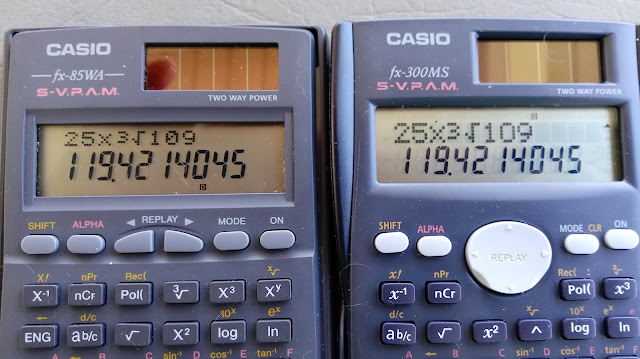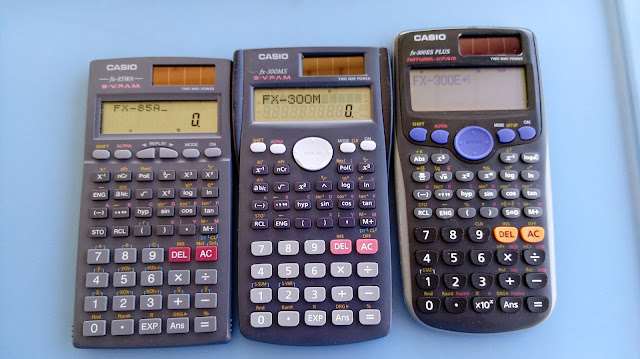Saturday, January 19, 2019

Retro Review: Casio fx-85WA

Retro Review: Casio fx-85WA

I want to give a special thank you to John Cadick. Cadick requested that I review this calculator and has loaned me his calculator to review. Much appreciated, and John, I hope you like this review.

General Information

Company: Casio
Type: Scientific – Algebraic (Perfect Algebraic Method)
Display: 10 digits with 2 digit exponents
Power: Solar with battery backup (LR44)
Memory Registers: 8: A, B, C, D, E, F, X, Y ,M
Years: Circa 1998-1999
Original Cost: \$10 to \$20, depending on the store (at the time)
Documentation: Manual, Quick Reference card
Two Line Display and Fractions

The Casio fx-85WA has a two line display: the top line for the mathematical expression to be evaluated, and the bottom line displays the answers. The font on the bottom line is bigger than the font on the top line. Later models will have the fonts of both lines of about equal size.Screen for fx-85WAS (left) and FX-300MS (right)

You can enter equations as they are written, no need to worry about post-fix notation. The order of operations is effect for all calculations.

You can enter and do calculations with fractions with the [ a b/c ] key. After a calculation is completed, the [ a b/c ] can convert results between a fraction and decimal approximation. All fractions are simplified. The maximum denominator is 9999. Conversions between mixed fractions and improper fractions are also available.

The functions available are:

* Trigonometry: sine, cosine, tangent, with inverse and hyperbolic
* Logarithmic: natural and common, with inverses
* Square, square root, cube, and cube root (all primary key functions on the fx-85WA)
* Reciprocal, factorial (calculating 69! on the fx-85WS is surprising slightly faster than later models)
* Combination, permutation
* Engineering notation with the [ENG] and its inverse
* Degree-Minute-Seconds calculations [ ° ‘ “ ] and conversions
* Fractions, random numbers, round result to the FIX settings
* Polar and Rectangular Conversions

For the polar and rectangular conversions, results are stored in the following variables:

E: x, r
F: y, θ

Replay

The last expression can be edited. To re-edit the last expression, simply press either the arrow keys [ ← ] or [ → ] and edit. Characters can be inserted and deleted.

Statistics

There are two primary statistics modes on the fx-85WA:

* SD (Single Deviation, 1-variable statistics)
* REG (Regression, 2-variable statistics)

The type of regressions are:

Lin: Linear (y = a + b*x)
Log: Logarithmic (y = a + b*ln x)
Exp: Exponential (y = a + b*e^x)
Pwr: Power (y = a*x^b)
Inv: Inverse (y = a + b/x)

Data points are entered with the [ M+ ] key with frequencies added with the semicolon ( ; ). When data is entered, the x value is returned. There is no data count indicator when [ M+ ] is pressed, I wish it did. Keep this in mind.

All the statistics registers are kept as shifted functions and alpha registers, and they are:

 SHIFT 1: mean x SHIFT 6: sy* RCL A: ∑x^2 RCL F: ∑xy SHIFT 2: σx SHIFT 7: A (intercept) RCL B: ∑x RCL M: ∑x^3 SHIFT 3: sx* SHIFT 8: B (slope, x coef.) RCL C: n RCL X: ∑x^2y SHIFT 4: mean y SHIFT 9: C (x^2 coef.) RCL D: ∑y^2 RCL Y: ∑x^4 SHIFT 5: σy SHIFT (: r (correlation) RCL E: ∑y

* labeled xσn-1 and yσn-1, respectively. (sample deviation)

Keyboard

The fx-85WA is a light calculator and pretty compact. The keys are pretty solid, but also allow for fast typing. I like the labeling of the keys, they are easy to read.
One thing I am big fan of both STO (store) and RCL (recall) are primary key functions. This is something we don’t see on scientific calculators anymore, as often either STO or RCL is a shifted function.

The color of the font on the keys and labels are readable with good contrast. The fx-85WA is a pleasure to use.

Verdict

The fx-85WA serves a scientific calculator providing with lots of functions. My only wish I had for this model is that top line’s font is bigger, but that is addressed in later models.

This model (and equivalent fx-300W) is a challenge to find. I searched ten pawn shops and several thrift shops for this model without success.

Before I go: I want to thank John Cadick for lending me his calculator to review.

I also want to give a table comparison of three Casio calculators:(left to right) Casio fx-85WA, Casio fx-300MS, Casio fx-300ES Plus

Eddie

All original content copyright, © 2011-2019. Edward Shore. Unauthorized use and/or unauthorized distribution for commercial purposes without express and written permission from the author is strictly prohibited. This blog entry may be distributed for noncommercial purposes, provided that full credit is given to the author. Please contact the author if you have questions.

1.2.